# Electric Current Exam 1 and Problem Solutions

1. Voltage vs. current graph of a conductor is given below. Find the change in the resistance of conductor in first and third intervals.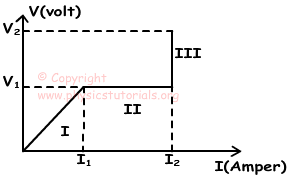We use ohm’s law to find relation between V, I and R.

Interval I: Since potential and current increase linearly, resistance of the conductor becomes constant.

Interval II: In this interval, potential is constant but current increases. Thus, resistance of the conductor must decrease to make potential constant.

Interval III: In this interval potential increases but current is constant. Thus, resistance of the conductor must increase to make potential increase.

2. a) Find the equivalent resistance of the circuit given below.

b) Find current passing through the circuit.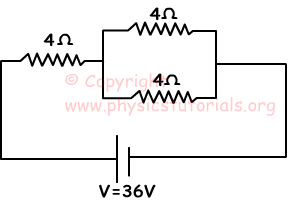a) Since resistance are connected in parallel between points A and B;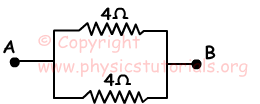1/Req=1/4+1/4

Req=2Ω

Equivalent resistance between points C and B;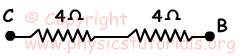Req=4+2=6Ω

b) V=I.R

36=I.6

I=6Amperes

3. a) Find equivalent resistance between the points X and Y.

b) If the current passing from 7Ω resistor is I₁, and current passing from 8Ω resistor is I₂, find I₁/I₂.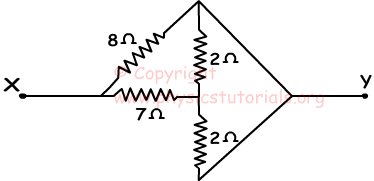a) We redraw circuit and make it simple;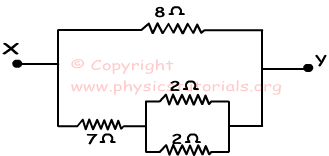Req=7+(2.2)/(2+2) (resistance in lower branch)

Req=8Ω

Equivalent resistance between points X and Y;

Req=(8.8)/(8+8)=4Ω

b)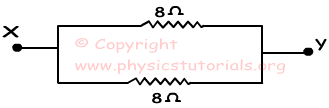Since resistances are in parallel, their potentials are equal. Using ohm’s law;

V₁=I₁.R₁ (lower branch)

V=I₁.8

V₂=I₂.R₂ (upper branch)

V=I₂.8

I₁/I₂=1

4. If we close switch shown in the picture given below, find the changes in the brightness of the bulbs.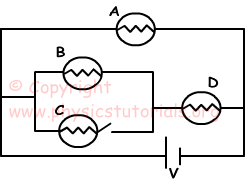Circuit when switch is closed;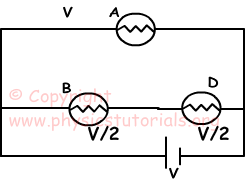Potential of B is equal to potential of D.

VA>VB=VD

When we close switch;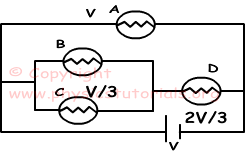potentials of the bulbs become;

VA=V

VB=VC=V/3

VD=2V/3

Thus; there is no change in the brightness of the bulb A, brightness of B decreases, and D increases.

5. Find the efficiency of the motor in the circuit given below.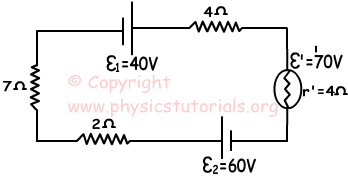Current passing through circuit is;

I=(ε₁+ε₂-ε’)/Req

I=(40+60-70)/(7+2+2+4)

I=2 Amperes

Efficiency=ε’/(ε’+I.r’)=70/(70+2.2)

Efficiency=35/37